Apply matched filtering using raised cosine FIR filter

•Libraries:
Communications Toolbox / Comm Filters
Communications Toolbox HDL Support / Comm Filters

## Description

The Raised Cosine Receive Filter block filters the input signal using a raised cosine finite impulse response (FIR) filter and optionally decimates the filtered signal. The FIR filter has (Filter span in symbols × Input samples per symbol + 1) tap coefficients. The block icon shows the impulse response of the filter. For more information, see Algorithms.

## Examples

expand all

The Raised Cosine Transmit Filter and Raised Cosine Receive Filter blocks are designed for raised cosine (RC) filtering. Each block can apply a square-root raised cosine (RRC) filter or a raised cosine filter to a signal. You can vary the roll-off factor and span of the filter.

The Raised Cosine Transmit Filter and Raised Cosine Receive Filter blocks are tailored for use at the transmitter and receiver, respectively. The transmit filter upsamples (interpolates) the output signal. The receive filter expects its input signal to be upsampled and downsamples (decimates) the output signal, based on the configured settings of the block.

The raised cosine transmit and receive filter blocks each introduce a propagation delay, as described in Group Delay.

The `doc_rrcfiltercompare.slx` model shows how to split the filtering equally between the transmitter and the receiver by using a pair of square root raised cosine filters. The use of a matched pair of square root raised cosine filters is equivalent to a single normal raised cosine filter. The filters share the same span and use the same number samples per symbol but the two filter blocks on the upper path have a square root shape and the single filter block on the lower path has the normal shape.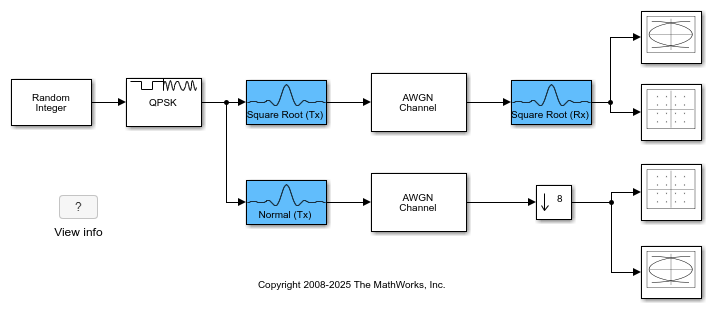Run the model and observe the eye and constellation diagrams. The performance is nearly identical for the two methods. Note that the limited impulse response of practical square root raised cosine filters causes a slight difference between the response of two cascaded square root raised cosine filters and the response of one raised cosine filter.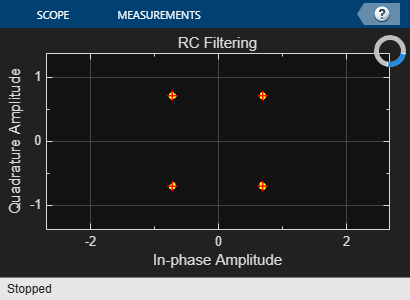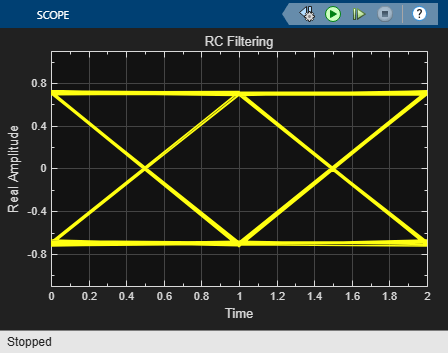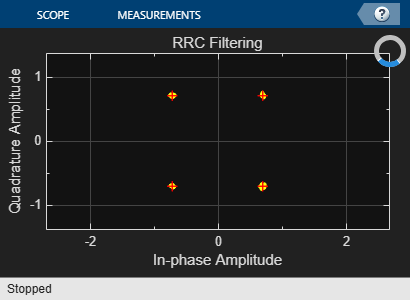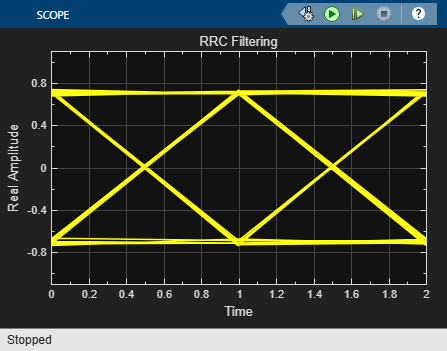Apply raised cosine matched filtering to a modulated signal by applying transmit and receive root raised cosine (RRC) filtering to the input signal. Demonstrate input signal power adjustment for correct SNR and the delay adjustment for correct error rate calculation.

The `cm_tx_rx_rrc_filter` model outputs frames of random integers by using a Random Integer Generator block. The frames of data pass through a QPSK Modulator Baseband block, Raised Cosine Transmit Filter, AWGN Channel block, Raised Cosine Receive Filter, and QPSK Demodulator Baseband block. Separate Error Rate Calculation blocks compute the bit error rate after QPSK demodulation, with and without accounting for the delay of the recieved signal due to the transmit and receive filtering.

The model initializes the variables that configure block parameters by using the `PreLoadFcn` callback function. For more information, see Model Callbacks (Simulink). The model demonstrates configuration of parameters to set the input signal power level for AWGN based on filter oversampling, and to set the delay for the error rate calculation path based on the filter delay.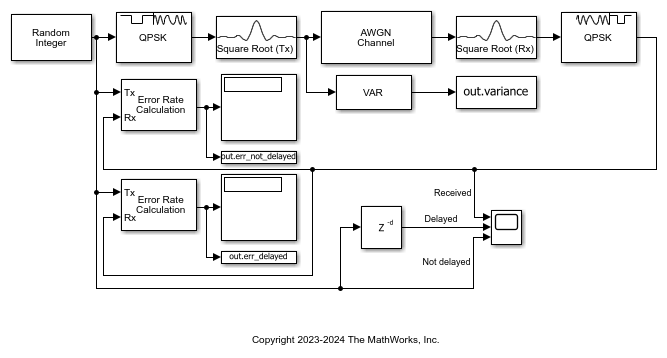The QPSK-modulated signal has unity power, but signal oversampling by the transmit RRC filtering reduces the signal level by the oversampling rate. To apply the correct noise level, you must adjust the input signal power in the AWGN block to account for the reduced signal level due to oversampling by the filter. A Variance block computes a variance to be approximately one over the oversampling rate, 1/8.

``` Computed variance = 0.1250 ```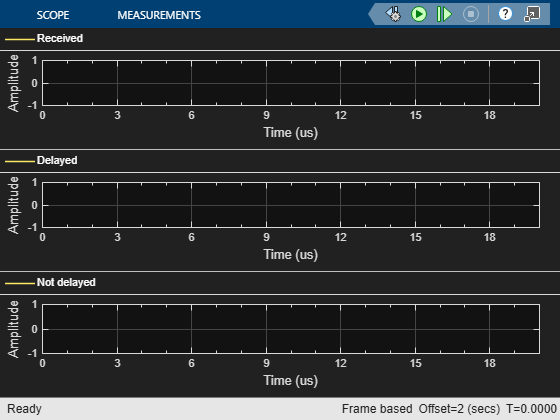A Time Scope plots the Received, Delayed, and Not delayed signals. The Delayed signal aligns with the Received signal, but the Not delayed signal is shifted by 10 samples. The error rate calculation shows the correct BER when you account for the filter delay.

``` Delay not accounted for: BER = 0.75 Delay accounted for: BER = 0.00458 ```

Recover frame synchronization from a QPSK system impaired by a variable timing error. The example shows the benefit of performing symbol synchronization to assist the frame synchronization.

The `cm_ex_symbol_frame_sync` model restores frame synchronization due to a variable timing error. The Variable Fractional Delay block introduces a varying timing error to a root raised cosine (RRC) filtered QPSK signal. After the AWGN block, the receive path is duplicated to compare performance with and without a Symbol Synchronizer block in the path. The Symbol Synchronizer block corrects for clock skew between the transmitter and receiver, aligning the output signal with a valid clock reference. For the timing error conditions in this example, the symbol synchronizer returns a vector containing 99, 100, or 101 symbols for a 200-sample input vector.

The Frame Synchronizer block aligns the symbol stream along correct frame boundaries using the frame header present in the signal. It also provides the valid frame indicator signal to the BER Data Decoding subsystem, which calculates the bit error rate (BER). To avoid calculating the BER on nonvalid frames, the BER Data Decoding subsystem regenerates the input data bits rather than using the Bit Generation block output.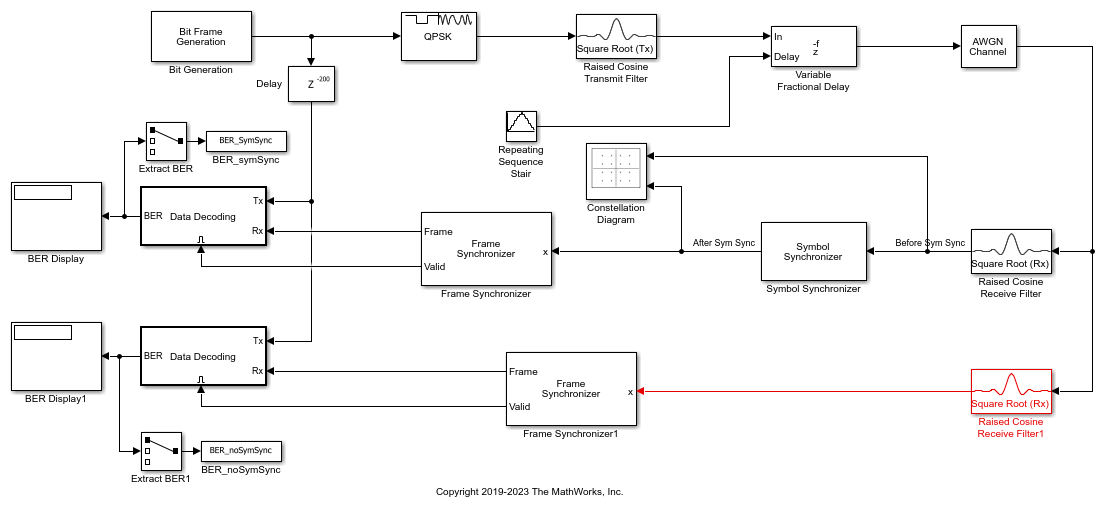For a 20 dB signal-to-noise ratio and variable timing error in the range of [0, 0.9] samples, signal recovery is successful on the receiver path that includes symbol synchronization. The timing error varies over time, causing the constellation to oscillate between corrupted and clean states as seen in the Before Sym Sync signal in the Constellation Diagram block. The After Sym Sync signal in the constellation diagram shows that the symbol synchronizer removes the variable timing error signal impairment.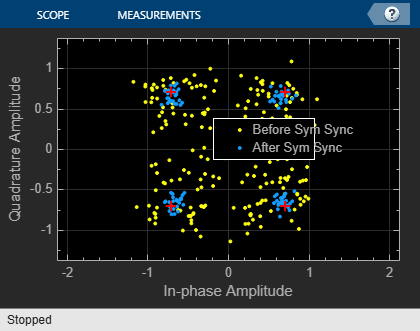The BER with and without symbol synchronization show the performance improvement due to the Symbol Synchronizer block.

```Error rate with symbol synchronization: 0.000 Error rate without symbol synchronization: 0.010 ```

## Ports

### Input

expand all

Input signal, specified as a column vector or a Ki-by-N matrix. Ki is the number of input samples per signal channel, and N is the number of signal channels.

For a Ki-by-N matrix input, the object processes columns of the input matrix as N independent channels.

Data Types: `single` | `double` | `fixed point`

### Output

expand all

Output signal, returned as a column vector or a Ko-by-N matrix. Ko is equal to Ki / Decimation factor. Ki is the number of input samples per signal channel, and N is the number of signal channels.

The block filters each channel over time and generates a Ko-by-N output matrix. The output signal is the same data type as the input signal.

Data Types: `single` | `double` | `fixed point`

## Parameters

expand all

To edit block parameters interactively, use the Property Inspector. From the Simulink Toolstrip, on the Simulation tab, in the Prepare gallery, select .

Main

Filter shape, specified as `Square root` or `Normal`. For more information, see Filter Characteristics.

Roll-off factor of the filter, specified as a scalar in the range [`0`, `1`].

Filter span in symbols, specified as an even positive integer. The block truncates the infinite impulse response length of the ideal raised cosine filter to an FIR with a length equal to the value of this parameter.

Number of input samples per symbol, specified as an integer value greater than `1`. This parameter defines the number of input samples that represent one symbol in the input signal.

Decimation factor the block applies to the input signal, specified as a positive integer. The output samples per symbol equals the value of the input samples per symbol divided by the decimation factor. If the decimation factor is one, then the block only applies filtering and there is no decimation.

Decimation offset in samples, specified as an integer in the range [`0`, (Decimation factor`1`).

Linear filter gain, specified as a positive scalar. The block scales the normalized filter coefficients by the linear filter gain value that you specify.

Input processing control, specified as one of these options:

• `Columns as channels (frame based)` — The block treats each column of the input as a separate channel.

• `Elements as channels (sample based)` — The block treats each element of the input as a separate channel.

Block processing rate for input signal downsampling and filtering, specified as one of these options:

• `Enforce single-rate processing` — When you select this option, the block maintains the input sample rate and processes the signal by decreasing the output frame size by a factor of K.

• `Allow multirate processing` — When you select this option, the block processes the signal such that the output sample rate is K times slower than the input sample rate.

#### Dependencies

To use `Enforce single-rate processing`, you must set Input processing to ```Columns as channels (frame based)```.

Select this parameter to save the filter coefficients as the MATLAB® workspace variable that you specify in the Coefficient variable name parameter.

Name of coefficient variable to create in the MATLAB workspace.

#### Dependencies

This parameter appears when you select Export filter coefficients to workspace.

To analyze the raised cosine filter response, click the View Filter Response button. MATLAB opens the Filter Visualization Tool, `FVTool`. When you change the block mask parameter settings, click the button again to open a new instance of `FVTool` and see the new filter characteristics. Previous instances of `FVTool` remain open and reflect your previous mask settings. Instances of `FVTool` remain open after you close the model.

Data Types

Select the rounding mode for fixed-point operations. The block uses rounding mode when the result of a fixed-point calculation does not map exactly to a number representable by the data type. The filter coefficients do not obey this parameter; they always round to `Nearest`. For more information, see Rounding Modes or Rounding Mode: Simplest (Fixed-Point Designer).

Select the overflow mode for fixed-point operations. The filter coefficients do not obey this parameter; they are always saturated.

Specify the word length and the fraction length of the filter coefficients (numerator and denominator).

This block is a subsystem that contains a FIR Decimation block. For diagrams showing the use of the coefficient data types in this block, see the filter structure diagrams for the Discrete FIR Filter (Simulink) block.

When you select `Inherit: Same word length as input`, the word length of the filter coefficients match that of the input to the block. In this mode, the fraction length of the coefficients is automatically set to the binary-point only scaling that provides you with the best precision possible given the value and word length of the coefficients.

The filter coefficients do not obey the Rounding mode and the Saturate on integer overflow parameters; they are always saturated and rounded to `Nearest`.

For information about specifying data types, see Data Type Assistant.

Specify the product output word and fraction lengths. For diagrams showing the use of the product output data type in this block, see the filter structure diagrams for the Discrete FIR Filter (Simulink) block and Multiplication Data Types.

When you select `Inherit: Same as input`, these characteristics match those of the input to the block.

For information about specifying data types, see Data Type Assistant.

Specify the accumulator word and fraction lengths. For diagrams showing the use of the accumulator data type in this block, see the filter structure diagrams for the Discrete FIR Filter (Simulink) block and Multiplication Data Types.

• When you select ```Inherit: Same as input```, these characteristics match those of the input to the block.

• When you select ```Inherit: Same as product output```, these characteristics match those of the product output.

For information about specifying data types, see Data Type Assistant.

Specify the output word and fraction lengths.

• When you select ```Inherit: Same as input```, these characteristics match those of the input to the block.

• When you select ```Inherit: Same as accumulator```, these characteristics match those of the accumulator.

For information about specifying data types, see Data Type Assistant.

Select this parameter to prevent the fixed-point tools from overriding the data types that you specify in the block dialog box. For more information, see the Fixed-Point Tool (Fixed-Point Designer).

## Block Characteristics

 Data Types `double` | `fixed pointa` | `single` Multidimensional Signals `no` Variable-Size Signals `yes` a Fixed-point outputs must be signed.

expand all

## Tips

Exporting Filter Coefficients to the MATLAB Workspace

• To examine or manipulate the coefficients of the filter that this block designs, select Export filter coefficients to workspace. Set the Coefficient variable name parameter to the name of a variable that you want the block to create in the MATLAB workspace. Running the simulation causes the block to create the variable, overwriting any previous contents in case the variable already exists.

## Algorithms

expand all

Characteristics of the raised cosine receive filter are the same as in the Raised Cosine Transmit Filter block, except that for the receive filter, the input response length of the filter depends on the value of the Input samples per symbol parameter.

## Version History

Introduced before R2006a